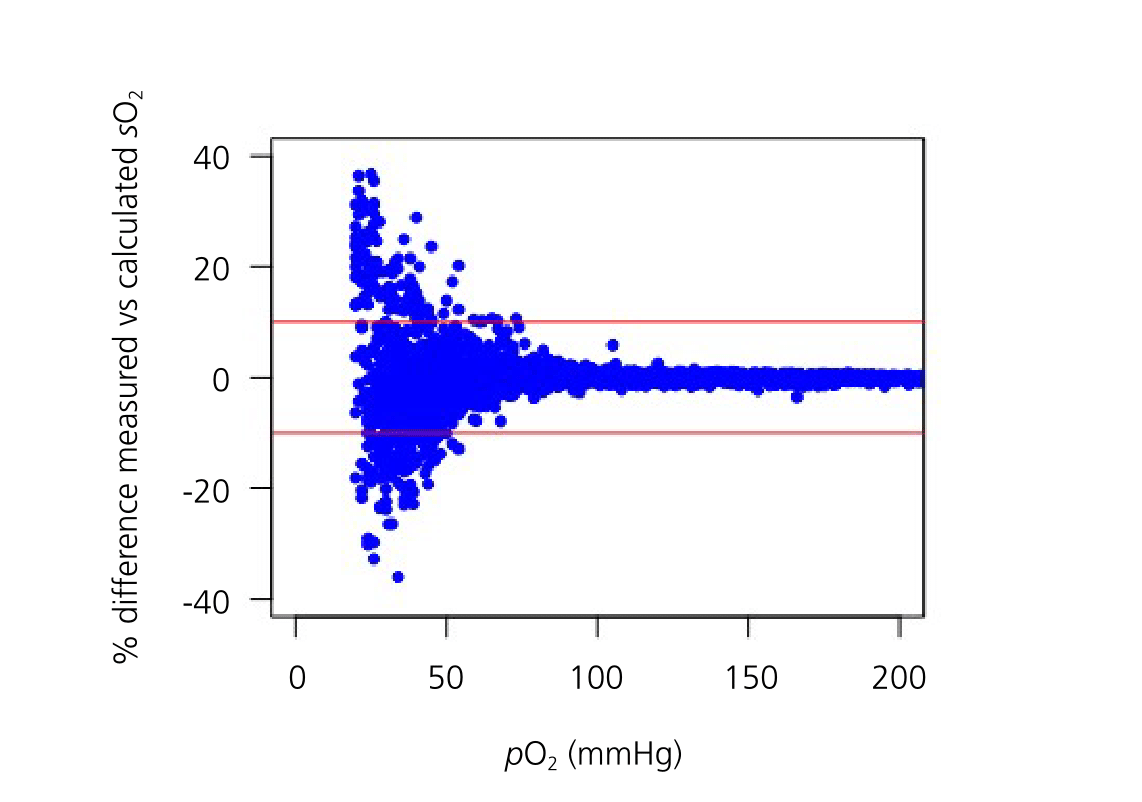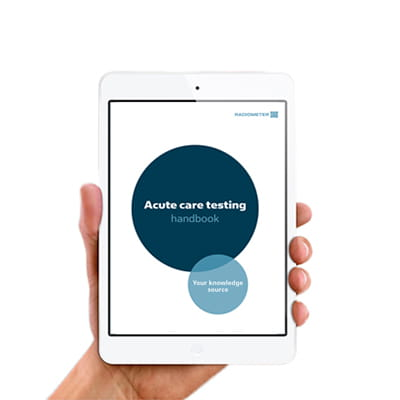Topics
Parameters

Article

April 2018

# Observation of clinically significant errors in oxygen saturation calculations when pO2 is low

Summary Calculation-based approaches to determining oxygen saturation, as used in some point-of-care tests, increasingly deviate from values measured directly by CO-oximetry as the partial pressure of oxygen in the blood decreases. Oxygen saturation should be measured by CO-oximetry when inaccuracies in calculations may negatively impact patient care.

This article addresses the accuracy of calculated oxygen saturation (sO2), as used in some point-of-care-testing analyzers, compared to direct measurements by CO-oximetry. Measured and calculated sO2 values were compared for 1180 patients. Discrepancies between measured and calculated sO2  ≥10 % were observed in 8 % of cases. Of these discrepant measurements, 94 % occurred when the partial pressure of oxygen (pO2) was less than 50 mmHg.

These results suggest that the potential for clinically significant error in sO2 calculations increases as pO2 decreases. When pO2 is low, sO2 should be measured directly by CO-oximetry to avoid potential errors in calculated values.

Summarized from: Gunsolus IL et al. Low pO2 contributes to potential error in oxygen saturation calculations using a point of care assay. Am J Clin Pathol 2017; 149: 82-86.

Oxygen saturation (sO2) is routinely monitored to assess respiratory status and to calculate other indicators of cardiac and respiratory status, such as global oxygen demand. Three primary methods are used to determine sO2: pulse oximetry, CO-oximetry, and calculations.

Pulse oximetry is used for non-invasive, real-time monitoring of sO2, while both CO-oximetry and calculations are used to quantify sO2 in blood samples. Direct measurement of sO2 by CO-oximetry is performed by many blood gas analyzers, while calculation-based approaches using mathematical models that relate sO2 to other measured blood parameters are used in some point-of-care (POC) tests without CO-oximetry.

A 2014 acutecaretesting.org article by Chris Higgins asserted that sO2 is better measured by CO-oximetry than calculated, since direct measurements avoid errors that can arise from the physiologic assumptions made by calculated methods. As stated previously, these errors can be propagated through to parameters derived from sO2, such as global oxygen demand and Fick’s cardiac output [1-4].

While the proposed superiority of measured over calculated sO2 is based on sound analytical arguments, there has to date been limited evidence directly supporting this assertion. This article presents evidence of discordance between measured and calculated sO2 that is particularly pronounced when the partial pressure of oxygen (pO2) is low.

### Index case spurs investigation

Our investigation of measured and calculated sO2 was motivated by a heart catheterization case in our hospital. In this case, a patient was observed to be hypoxic when sO2 was measured by CO-oximetry. The severity of hypoxemia (sO2 = 37 %, see Table I) prompted the physician to check this value against a second value obtained using a calculated sO2 from a POC test (sO2 = 55 %). The measured (CO-oximetry) and calculated (POC) sO2 values were noted to be significantly discrepant; this discrepancy was also observed when paired tests were performed approximately one hour later.

 Time sO2 (%) Method 12:27 37 CO-oximetry (measured) 12:32 55 POC (calculated) 13:23 39 CO-oximetry (measured) 13:24 59 POC (calculated)

TABLE I: sO2 values measured by CO-oximetry or calculated by a POC device during a catheterization procedure.

### Comparing measured and calculated sO2

We began to investigate the source of this discrepancy by comparing measured and calculated sO2 across many patients. We first accessed 3323 archived sO2 values from our laboratory’s CO-oximeter in 1180 patients. We then used the mathematical model employed by our institution’s POC test (Equation 1) to calculate the sO2 that would be reported by the POC test for each patient. The necessary inputs to this equation (pO2, pH, and bicarbonate concentration, the latter derived from pCO2) were measured by the CO-oximeter simultaneously with sO2 and so were available in the instrument archives.

Equation 1:

$$\textit{s}o_2 = {x^3 +150x \ \over x^3+150x+23400},$$
where x = pO2⋅100.48⋅(pH−7,4)−0.0013⋅([HCO3-]−25)

Using this procedure, we obtained paired values of measured and calculated sO2 for each patient. We then calculated the percent difference between these paired values to assess the expected agreement between the CO-oximeter and the POC test.

To assess the validity of our approach, we applied it to the index case in which sO2 was measured using both CO-oximeter and POC testing at approximately the same time. We calculated that the POC test would report an sO2 of 48 %, compared to the value of 55 % that was observed. This demonstrates that our approach provides a reasonable estimate of the agreement between the CO-oximeter and the POC test.

The results of our analysis showed that 8 % of calculated sO2 values were ≥10 % different from their paired measured value; we refer to these cases as discrepant. Over- and underestimation of the measured value occurred with approximately equal frequency. Notably, the majority of discrepant cases (94 %) occurred when pO2 was less than 50 mmHg. As shown in Fig. 1, the frequency of discrepancies between measured and calculated sO2 increased with decreasing pO2FIG. 1: Agreement between measured and calculated sO2 values as a function of pO2. Figure reproduced from Gunsolus IL et al. Low pO2 contributes to potential error in oxygen saturation calculations using a point of care assay, Am J Clin Pathol 2017; 149: 82-86.

The frequency of discrepancies did not depend significantly on either the pH or bicarbonate concentration. However, the distribution of pH and bicarbonate concentration did shift to lower values in discrepant vs. non-discrepant cases.

The frequency of pH ≤7.4 was 16 % higher in discrepant cases than in non-discrepant cases, and the frequency of bicarbonate concentration ≤25 mEq/L was 3 % higher. These results suggest that discrepancies between measured and calculated sO2 are more likely in patients whose blood parameters deviate significantly from normal, particularly when pO2 is less than 50 mmHg.

### Potential source of discrepancies

We observed that sO2 values calculated using the model employed by a POC instrument increasingly diverge from sO2 values measured by CO-oximetry as pO2 decreases. Deviations of pH and bicarbonate concentration from physiologic normal were also more common in discrepant vs. non-discrepant cases, though these parameters did not serve as independent predictors of discrepancies.

Precedent studies using smaller sample sets have also observed deviations of sO2 calculations from measured values under some conditions. One study observed consistently lower calculated than measured sO2 values in hypoxic blood samples, where pO2 was set to 10 or 20 mmHg using tonometry . Another study showed that calculated sO2 values in 21 critically ill patients increasingly diverged from measured values with decreasing pO2 .

Our study did not directly assess the mechanism responsible for the increasing deviation of calculated sO2 from measured sO2 as pO2 decreases. However, we suggest that these deviations result in part from the increasing steepness of the O2-hemoglobin dissociation curve as pO2 decreases, together with inter-individual variability in hemoglobin-O2 dissociation.

While sO2 calculations assume normal hemoglobin-O2 dissociation behavior, a precedent study has shown that inter-individual variability in hemoglobin-O2 dissociation can lead to significant variation in measured sO2 at a given pO2 (i.e., sO2 ranged from 70-99 % when pO2 was 60 mmHg) . Deviations from normal may therefore introduce error to sO2 calculations.

This error is expected to be greater at low pO2, where the O2-hemoglobin dissociation curve is more sensitive to errors in pO2 measurement due to its greater slope.

### Using measured and calculated sO2 in clinical practice

Our observations are most relevant to clinicians who treat critically ill patients using POC sO2 testing employing calculation-based approaches. We recommend that sO2 be measured by CO-oximetry, rather than calculated, in patients who are expected to have low pO2 or anytime calculated sO2 appears inconsistent with the clinical picture.

Clinicians cannot expect to correct calculated sO2 values using a simple correction procedure, since positive and negative deviations from measured values occur with similar frequency. Overall, we recommend that sO2 be directly measured by CO-oximetry in settings where inaccuracies in sO2 calculations may have significant consequences on patient care.

References
1. Woda RP, Dzwonczyk RD, Orlowski JP et al. Effect of measurement error on calculated variables of oxygen transport. J Appl Physiol 1996; 80: 559-63.
2. Myburgh JA. Derived oxygen saturations are not clinically useful for the calculation of oxygen consumption. Anaesth Intensive Care 1992; 20: 460-63.
3. Johnson PA, Bihari DJ, Raper RF et al. A comparison between direct and calculated oxygen saturation in intensive care. Anaesth Intensive Care 1993; 21: 72-75.
4. Hess D, Elser RC, Agarwal NN. The effects on the pulmonary shunt value of using measured versus calculated hemoglobin oxygen saturation and of correcting for the presence of carboxyhemoglobin and methemoglobin. Respir Care 1984; 29: 1001-05.
5. Porath M, Sinha P, Dudenhausen JW et al. Systematic instrumental errors between oxygen saturation analysers in fetal blood during deep hypoxemia. Clin Chim Acta 2001; 307: 151-57.
6. Gøthgen IH, Siggaard-Andersen O, Kokholm G. Variations in the hemoglobin-oxygen dissociation curve in 10079 arterial blood samples. Scand J Clin Lab Investig Suppl 1990; 203: 87-90.
References
1. Woda RP, Dzwonczyk RD, Orlowski JP et al. Effect of measurement error on calculated variables of oxygen transport. J Appl Physiol 1996; 80: 559-63.
2. Myburgh JA. Derived oxygen saturations are not clinically useful for the calculation of oxygen consumption. Anaesth Intensive Care 1992; 20: 460-63.
3. Johnson PA, Bihari DJ, Raper RF et al. A comparison between direct and calculated oxygen saturation in intensive care. Anaesth Intensive Care 1993; 21: 72-75.
4. Hess D, Elser RC, Agarwal NN. The effects on the pulmonary shunt value of using measured versus calculated hemoglobin oxygen saturation and of correcting for the presence of carboxyhemoglobin and methemoglobin. Respir Care 1984; 29: 1001-05.
5. Porath M, Sinha P, Dudenhausen JW et al. Systematic instrumental errors between oxygen saturation analysers in fetal blood during deep hypoxemia. Clin Chim Acta 2001; 307: 151-57.
6. Gøthgen IH, Siggaard-Andersen O, Kokholm G. Variations in the hemoglobin-oxygen dissociation curve in 10079 arterial blood samples. Scand J Clin Lab Investig Suppl 1990; 203: 87-90.
Disclaimer

May contain information that is not supported by performance and intended use claims of Radiometer's products. See also Legal info.Ian Gunsolus

PhD, Clinical chemistry fellow,

Hennepin County Medical Center,

Minneapolis, MN, USA

Articles by this author## Get the acute care testing handbook

Your practical guide to critical parameters in acute care testing.